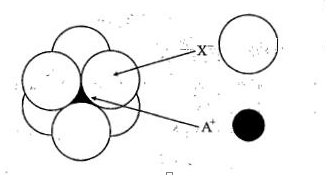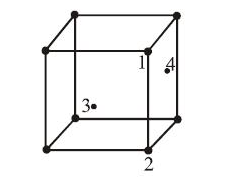# NEET Practice Questions - Chemistry - The Solid State• Subject:

Chemistry

• Chapter:

The Solid State

If NaCl is doped with 10-4 mol % of SrCl2, the concentration of cation vacancies will be (NA = 6.023 x 1023 mol-1)

(a) 6.023 x 1015 mol-1                                            (b) 6.023 x 1016 mol-1

(c) 6.023 x 1017 mol-1                                            (d) 6.023 x 1014 mol-1

CsCl crystallises in body-centred cubic lattice. If 'a' is its edge length then which of the following expressions is correct?

(a) rCs+ + rCl- = 3a

(b)  rCs+ + rCl- = 3a/2

(c)  rCs+ + rCl- = ($\sqrt{3}$/2)*a

(d) rCs+ + rCl-$\sqrt{3a}$

The phenomenon in which polar crystals on heating produce electricity is called:-
(a) pyro-electricity
(b) piezo-electricity
(c) ferro-electricity
(d) ferri-electricity

The edge length of cube is 400pm. Its body diagonal would be-

(A) 500 pm
(B) 693 pm
(C) 600 pm
(D) 566 pm

Metals have conductivity of the order of (ohm-1 cm-1):-

(a)1012
(b)108
(c)102
(d)10-6

Total volume of atoms present in a face centred cubic unit cell of a metal is ( r is atomic radius):

(A) 20/3 $\pi$r3

(B)  24/3 $\pi$r3

(C)  12/3 $\pi$r3

(D)  16/3 $\pi$r3

A solid PQ have rock salt type structure in which Q atoms are at the corners of the unit cell. If the body centred atoms in all the units cells are missing, the resulting stoichiometry will be

(A) PQ

(B) PQ2

(C) P3Q4

(D) P4Q3

Lithium borohydride (LiBH4), crystallises in an orthorhombic system with 4 molecules per unit cell. The unit cell dimensions are:- a = 6.81 Å , b = 4.43 Å ,c = 717 Å. If the molar mass of LiBH4 is
21.76 g mol-1.The density of the crystal is:-

(A) 0.668 gcm-3
(B) 0.585 gcm2
(C) 1.23 gcm-3

(D) None of these

The arrangement of Xions around A+ ion in solid AX is given in the figure (not drawn to scale).
If the radius of X is 250 pm,the radius of A+ is:(a)104 pm
(b)125 pm
(c)183 pm
(d) 57 pm

In an f.c.c. unit cell. atoms are numbered as shown below.The atoms not touching each other are (Atom numbered 3 is face centre of front face).(A) 3&4
(B) 1&3
(C) 1&2
(D) 2&4